# The vaccination

The vaccination coverage of the population is 80%. Unvaccinated make up 60% of all infected. What percentage are unvaccinated more likely to be infected?

p =  40 %

### Step-by-step explanation: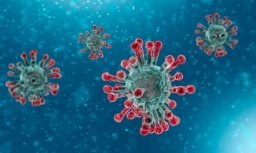Did you find an error or inaccuracy? Feel free to write us. Thank you!Tips to related online calculators
Would you like to compute count of combinations?

## Related math problems and questions:

• Double probabilityThe probability of success of the planned action is 60%. What is the probability that we will achieve success at least once if this action is repeated twice?
• The universityAt a certain university, 25% of students are in the business faculty. Of the students in the business faculty, 66% are males. However, only 52% of all students at the university are male. a. What is the probability that a student selected at random in the
• Alarm systems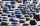What is the probability that at least one alarm system will signal the theft of a motor vehicle when the efficiency of the first system is 90% and of the independent second system 80%?
• Utopia IslandThe probability of disease A on the island of Utopia is 40%. The probability of occurrence among the men of this island, which make up 60% of all the population (the rest are women), is 50%. What is the probability of occurrence of A disease among women o
• Gym center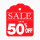80% of all visitors to the gym center enjoy a discount. 3/4 of all visitors go to practice regularly. All visitors who go to the gym regularly benefit from a discount. What percentage of all visitors do not go to the gym regularly but still use the discou
• Alcohol mixingHow much 55% alcohol we must pour into 1500 g 80% alcohol to form a 60% alcohol? How much 60% alcohol created?
• Lathe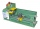95% of the components manufactured on the lathe comply with the standard, of which 80% of the components are first-class. How likely can we expect a manufactured part to be first class?
• SolutionWhat amount of 80% solution and what amount of water is needed to mix in order to obtain two liters of 60% solution of this mixture?
• Class - boys and girls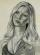In the class are 60% boys and 40% girls. Long hair has 10% boys and 80% girls. a) What is the probability that a randomly chosen person has long hair? b) The selected person has long hair. What is the probability that it is a girl?
• ConcentrationHow many grams of 65% and 50% acid is to be mixed to give 240 g acid with a concentration of 60%?
• Alcohol solutionsWe have to produce 2 liters of 60% alcohol from 55% and 80%. How many of which ones will we use in the solution?
• Alcohol mixtureFrom 55% and 80% alcohol, we have to produce 0.2 kg of 60% alcohol. How many of them do we use in the solution?
• SpiritFrom 55% and 80% spirit we would like to produce 0.2 kg of 60% spirit. How many of them we must use in a solution?
• Sick days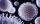In Canada, there are typically 261 working days per year. If there is a 4.9% chance that an employee takes a sick day. .. what is the probability an employee will use 17 OR MORE sick days in a year?
• Alcohol mixing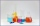How much 64% of the alcohol must be poured into 6 liters of 85% alcohol to produce 79% alcohol?
• MasteringThe student masters the subject matter for the exam in Czech to 98%, from Math to 86% and from Economics to 71%. What is the probability that he will fail from Math and from others will succeed?
• Left handedIt is known that 25% of the population is left-handed. What is the probability that there is a maximum of three left-handers at a seminar where there are 30 participants?